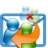# Format Telephone Numbers

## A sample for how to consistently format the phone numbers of your contacts.

Last modified: 2013/12/24 | Accessed: 33.271  | #103
◀ Previous sample Next sample ▶ReplyAll ReplyAll alerts you before unintentionally replying all, or if you are a confidential BCC recipient of the e-mail.

This example converts certain telephone and fax numbers to a unique format: '+Country Code (Area Code) Number'. See the FormatPhonenumbers_Ex function for which numbers are covered (BusinessTelephoneNumber, BusinessFaxNumber etc.).

At the top see the FormatPhonenumbers function, there the line m_DebugMode = True. This setting means the original number and the result of the conversion will be printed only to the debug window (ctrl+g). This way you can test whether or not the result would be correct. If you change that line to m_DebugMode = False and run the procedure again, the changes will be saved to the contacts.

In the procedure ParseNumber there is the constant Def_CountryCode, which holds the German country code by default. If the country code is missing for a number, this default code will be inserted. Change the value to your own country code if necessary.

Add a new module to the Outlook VBA project, and paste all the code. You can call it by pressing ALT+F8.How to add macros to Outlook
```Private m_DebugMode As Boolean

Public Sub FormatPhonenumbers()
Dim coll As VBA.Collection
Dim obj As Object
Dim Contact As Outlook.ContactItem
Dim IsInspector As Boolean

'If True, results will only be printed to the debug window (ctrl+g)
'If False, changes will be made to the contacts and saved.
m_DebugMode = True

Set coll = GetCurrentItems(IsInspector)
If coll.Count = 0 Then Exit Sub
For Each obj In coll
If TypeOf obj Is Outlook.ContactItem Then
Set Contact = obj
If m_DebugMode Then
Debug.Print "--" & vbCrLf & Contact.FileAs
End If
FormatPhonenumbers_Ex Contact
If m_DebugMode = False And IsInspector = False Then
If Contact.Saved = False Then Contact.Save
End If
End If
Next
End Sub

Private Sub FormatPhonenumbers_Ex(Contact As Outlook.ContactItem)
Dim cc\$, vw\$, no\$, OldNo\$, NewNo\$
Dim IsFax As Boolean

If Len(OldNo) Then
ParseNumber OldNo, cc, vw, no, IsFax
NewNo = JoinNumber(cc, vw, no, IsFax)
If m_DebugMode Then
Debug.Print OldNo: Debug.Print NewNo & vbCrLf
ElseIf OldNo <> NewNo Then
End If
End If

If Len(OldNo) Then
ParseNumber OldNo, cc, vw, no, IsFax
NewNo = JoinNumber(cc, vw, no, IsFax)
If m_DebugMode Then
Debug.Print OldNo: Debug.Print NewNo & vbCrLf
ElseIf OldNo <> NewNo Then
End If
End If

OldNo = Contact.CompanyMainTelephoneNumber
If Len(OldNo) Then
ParseNumber OldNo, cc, vw, no, IsFax
NewNo = JoinNumber(cc, vw, no, IsFax)
If m_DebugMode Then
Debug.Print OldNo: Debug.Print NewNo & vbCrLf
ElseIf OldNo <> NewNo Then
Contact.CompanyMainTelephoneNumber = NewNo
End If
End If

OldNo = Contact.HomeTelephoneNumber
If Len(OldNo) Then
ParseNumber OldNo, cc, vw, no, IsFax
NewNo = JoinNumber(cc, vw, no, IsFax)
If m_DebugMode Then
Debug.Print OldNo: Debug.Print NewNo & vbCrLf
ElseIf OldNo <> NewNo Then
Contact.HomeTelephoneNumber = NewNo
End If
End If

OldNo = Contact.MobileTelephoneNumber
If Len(OldNo) Then
ParseNumber OldNo, cc, vw, no, IsFax
NewNo = JoinNumber(cc, vw, no, IsFax)
If m_DebugMode Then
Debug.Print OldNo: Debug.Print NewNo & vbCrLf
ElseIf OldNo <> NewNo Then
Contact.MobileTelephoneNumber = NewNo
End If
End If
End Sub

Private Function JoinNumber(cc\$, vw\$, no\$, IsFax As Boolean) As String
Dim n\$
If IsFax Then n = "Fax: "
If Len(cc) Then n = n & cc
If Len(vw) Then vw = " (" & vw & ") "
n = n & vw
n = n & no
JoinNumber = n
End Function

Private Sub ParseNumber(ByVal Number\$, cc\$, vw\$, no\$, IsFax As Boolean)
Dim p1&, p2&
Dim i&
Const Def_CountryCode As String = "+49"

cc = "": vw = "": no = ""
Number = Trim\$(Number)

If LCase\$(Left\$(Number, 4)) = "fax:" Then
IsFax = True
Number = Trim\$(Mid\$(Number, 5))
Else
IsFax = False
End If

If Left\$(Number, 2) = "00" Then Number = "+" & Mid\$(Number, 3)
If Left\$(Number, 1) = "0" Then Number = Mid\$(Number, 2)
If Left\$(Number, 1) <> "+" Then
'Assuming the country code is missing, insert the own country code.
Number = Def_CountryCode & " " & Number
End If
Number = Replace(Number, "/", " ")
Number = Replace(Number, "-", " ")
Number = Replace(Number, "(0", "(")
Number = Replace(Number, "(", " ")
Number = Replace(Number, ")", " ")
While InStr(Number, "  ") > 0
Number = Replace(Number, "  ", " ")
Wend

If Left\$(Number, 1) = "+" Then
For i = 2 To Len(Number)
If IsNumeric(Mid\$(Number, i, 1)) = False Then
If p1 = 0 Then
p1 = i
ElseIf p2 = 0 Then
p2 = i
Else
Exit For
End If
End If
Next
If p1 = 0 Then
no = Number
Else
cc = Mid\$(Number, 1, p1 - 1)
If p2 Then
vw = Mid\$(Number, p1 + 1, p2 - p1 - 1)
no = Mid\$(Number, p2 + 1)
Else
no = Mid\$(Number, p1)
End If
End If
End If
End Sub

Private Function GetCurrentItems(Optional IsInspector As Boolean) As VBA.Collection
Dim coll As VBA.Collection
Dim Win As Object
Dim Sel As Outlook.Selection
Dim obj As Object
Dim i&

Set coll = New VBA.Collection
Set Win = Application.ActiveWindow

If TypeOf Win Is Outlook.Inspector Then
IsInspector = TrueReporter VBOffice Reporter is an easy to use tool for data analysis and reporting in Outlook. A single click, for instance, allows you to see the number of hours planned for meetings the next month.